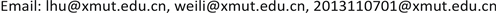1. 引言

2. 递归神经网络

Jordon在1986年第一次提出了递归神经网络，其网络结构如图1所示。

h k ( t + 1 ) = [ 1 + d T k ] h k ( T ) + d T k f ( Y k ( t ) ) (1)

Y k ( t ) = ∑ l = 1 n w k , l h l ( t ) + ∑ l = 1 m w k , l + n a l ( t ) (2)

E = 1 2 ∑ k = 1 n ( h k ( t ) − b k ( t ) ) 2 (3)

Δ w i j ( t + 1 ) = Z ∑ K = 1 N e k ( t + 1 ) L h k L w i j ( t + 1 ) (4)

L h k L w i j ( t + 1 ) = [ 1 + d T k ] L h k L w i j ( t ) + d T k L f ( S k ( t ) ) L S k × [ ∑ l = 1 n w k l ( t ) L h l L w i j ( t ) + δ k Z j ( t ) ] (5)

e ( t + 1 ) = { b k ( t + 1 ) − h k ( t + 1 )           若 k 为 输 出 神 经 元 0                                                             若 k 不 为 输 出 神 经 元 (6)

z j ( t ) = { h j ( t ) ,     j = 1 , 2 , ⋯ , n a j ( t ) ,     j = n + 1 , n + 2 , ⋯ , n + m (7)

W l k 为Kronecker delta函数

W l k = { 0       i ≠ k 1           i = k (8)

1) 给定初始权值，学习速度Z，惯量因子T，误差限值A和最大学习次数N；

2) 读入样本集{A(t), H(t)}；

3) 计算每一个互联神经元在(t + 1)时刻的输出；

4) 修正权值；

5) 计算误差E，若E小于给定误差限值A，则退出计算；否则进入步骤3重新学习。

3. 基于RNN的纳税评估模型

3.1. 纳税评估的背景

3.2. 预警指标选取

3.3. RNN模型构建3.3.1. 网络结构设计

The index data and codin
A1增值税税负率(累计)
A2增值税税负差异率(累计)
A3增值税税负变动率(累计同比)
A4销售毛利率(累计)
A5主营业务成本变动率(累计同比)
A6主营业务收入变动率(累计同比)
A7增值税税收贡献增长率(累计同比)
A8存货月周转率(累计平均)

n = ( n t + n 0 ) 2 3 + b (9)

h n = f [ ∑ j = 1 6 v j f ( ∑ i = 1 8 w j i + s j ) + s n ] ,     n = 1 , 2 , 3 (10)

3.3.2. 样本训练及结果检验

The index dat

10.03160.03980.13950.08360.05140.03260.166337.8562
20.03560.1025−0.08020.0815−0.3264−0.3776−0.427316.2031
30.02340.0728−0.03260.0591−0.3026−0.3006−0.31455.0216
40.07251.4225−0.35260.0789−0.4571−0.4487−0.5412208072
50.04850.27480.03560.1824−0.4562−0.4132−0.37454.2356
60.03700.2252−0.07730.0125−0.0920−0.0854−0.156246.5692
70.07051.4433−0.02080.1588−0.0245−0.0482−0.068914.1985
80.03120.0562−0.03120.09450.16990.19310.16018.3256
90.15624.52314.1265−0.1732−0.5236−0.65411.11393.2546
100.03080.01990.03560.09050.26540.23180.256813.4806

The contrast on inspection results and samples output

(0，0，1)(0.0001，0.0001，0.9992)可能性小
(0，1，0)(0.0011，0.9856，0.0000)可能性一般
(0，1，0)(0.0002，0.9992，0.0001)可能性一般
(0，0，1)(0.9879，0.0002，0.0001)可能性大误判
(0，0，1)(0.0086，0.0001，0.9968)可能性小
(0，0，1)(0.0011，0.0020，0.9945)可能性小
(1，0，0)(1.0000，0.0008，0.0025)可能性大
(1，0，0)(1.0000，0.0006，0.0023)可能性大
(0，1，0)(1.0000，0.0032，0.0001)可能性大误判
(1，0，0)(1.0000，0.0001，0.0007)可能性大
(1，0，0)(1.0000，0.0023，0.0002)可能性大
(1，0，0)(1.0000，0.0001，0.0026)可能性大

4. 实验分析

5. 结语